Anzeige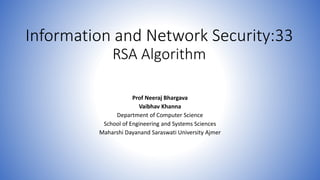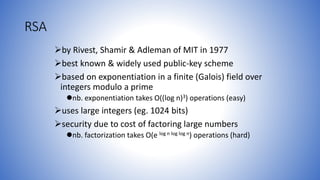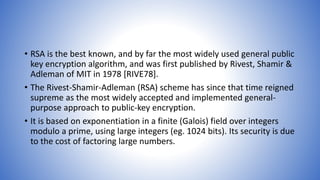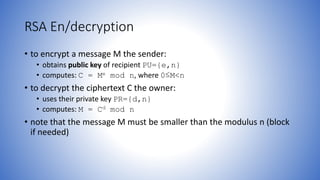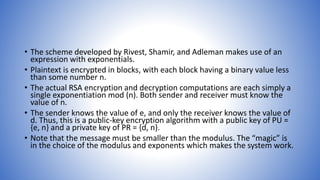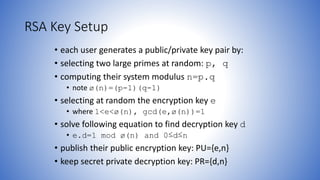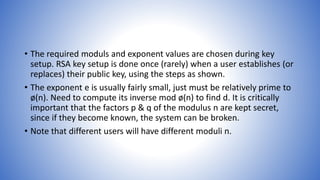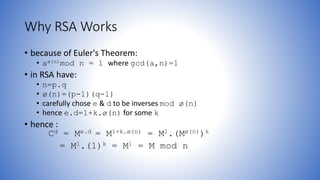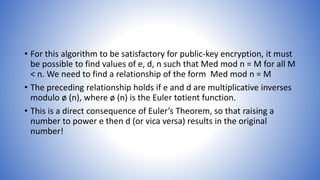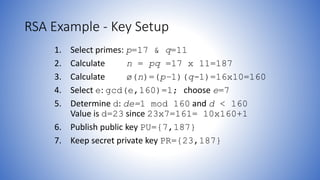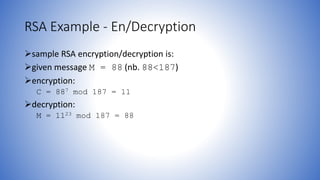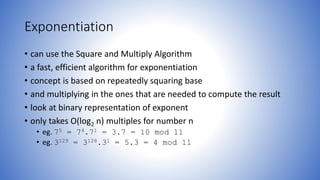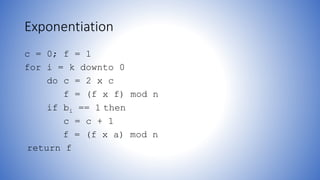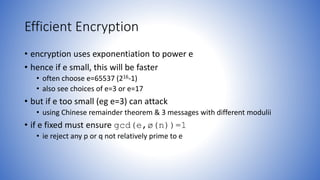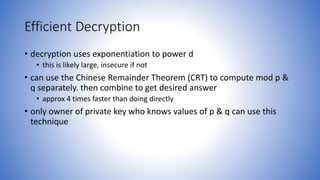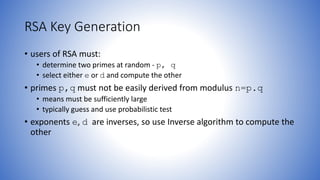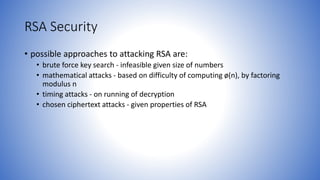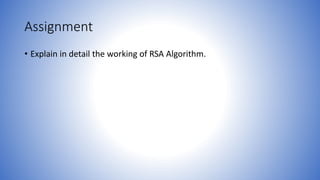1 von 18
Anzeige

### Information and network security 33 rsa algorithm

1. Information and Network Security:33 RSA Algorithm Prof Neeraj Bhargava Vaibhav Khanna Department of Computer Science School of Engineering and Systems Sciences Maharshi Dayanand Saraswati University Ajmer
2. RSA by Rivest, Shamir & Adleman of MIT in 1977 best known & widely used public-key scheme based on exponentiation in a finite (Galois) field over integers modulo a prime nb. exponentiation takes O((log n)3) operations (easy) uses large integers (eg. 1024 bits) security due to cost of factoring large numbers nb. factorization takes O(e log n log log n) operations (hard)
3. • RSA is the best known, and by far the most widely used general public key encryption algorithm, and was first published by Rivest, Shamir & Adleman of MIT in 1978 [RIVE78]. • The Rivest-Shamir-Adleman (RSA) scheme has since that time reigned supreme as the most widely accepted and implemented general- purpose approach to public-key encryption. • It is based on exponentiation in a finite (Galois) field over integers modulo a prime, using large integers (eg. 1024 bits). Its security is due to the cost of factoring large numbers.
4. RSA En/decryption • to encrypt a message M the sender: • obtains public key of recipient PU={e,n} • computes: C = Me mod n, where 0≤M<n • to decrypt the ciphertext C the owner: • uses their private key PR={d,n} • computes: M = Cd mod n • note that the message M must be smaller than the modulus n (block if needed)
5. • The scheme developed by Rivest, Shamir, and Adleman makes use of an expression with exponentials. • Plaintext is encrypted in blocks, with each block having a binary value less than some number n. • The actual RSA encryption and decryption computations are each simply a single exponentiation mod (n). Both sender and receiver must know the value of n. • The sender knows the value of e, and only the receiver knows the value of d. Thus, this is a public-key encryption algorithm with a public key of PU = {e, n} and a private key of PR = {d, n}. • Note that the message must be smaller than the modulus. The “magic” is in the choice of the modulus and exponents which makes the system work.
6. RSA Key Setup • each user generates a public/private key pair by: • selecting two large primes at random: p, q • computing their system modulus n=p.q • note ø(n)=(p-1)(q-1) • selecting at random the encryption key e • where 1<e<ø(n), gcd(e,ø(n))=1 • solve following equation to find decryption key d • e.d=1 mod ø(n) and 0≤d≤n • publish their public encryption key: PU={e,n} • keep secret private decryption key: PR={d,n}
7. • The required moduls and exponent values are chosen during key setup. RSA key setup is done once (rarely) when a user establishes (or replaces) their public key, using the steps as shown. • The exponent e is usually fairly small, just must be relatively prime to ø(n). Need to compute its inverse mod ø(n) to find d. It is critically important that the factors p & q of the modulus n are kept secret, since if they become known, the system can be broken. • Note that different users will have different moduli n.
8. Why RSA Works • because of Euler's Theorem: • aø(n)mod n = 1 where gcd(a,n)=1 • in RSA have: • n=p.q • ø(n)=(p-1)(q-1) • carefully chose e & d to be inverses mod ø(n) • hence e.d=1+k.ø(n) for some k • hence : Cd = Me.d = M1+k.ø(n) = M1.(Mø(n))k = M1.(1)k = M1 = M mod n
9. • For this algorithm to be satisfactory for public-key encryption, it must be possible to find values of e, d, n such that Med mod n = M for all M < n. We need to find a relationship of the form Med mod n = M • The preceding relationship holds if e and d are multiplicative inverses modulo ø (n), where ø (n) is the Euler totient function. • This is a direct consequence of Euler’s Theorem, so that raising a number to power e then d (or vica versa) results in the original number!
10. RSA Example - Key Setup 1. Select primes: p=17 & q=11 2. Calculate n = pq =17 x 11=187 3. Calculate ø(n)=(p–1)(q-1)=16x10=160 4. Select e: gcd(e,160)=1; choose e=7 5. Determine d: de=1 mod 160 and d < 160 Value is d=23 since 23x7=161= 10x160+1 6. Publish public key PU={7,187} 7. Keep secret private key PR={23,187}
11. RSA Example - En/Decryption sample RSA encryption/decryption is: given message M = 88 (nb. 88<187) encryption: C = 887 mod 187 = 11 decryption: M = 1123 mod 187 = 88
12. Exponentiation • can use the Square and Multiply Algorithm • a fast, efficient algorithm for exponentiation • concept is based on repeatedly squaring base • and multiplying in the ones that are needed to compute the result • look at binary representation of exponent • only takes O(log2 n) multiples for number n • eg. 75 = 74.71 = 3.7 = 10 mod 11 • eg. 3129 = 3128.31 = 5.3 = 4 mod 11
13. Exponentiation c = 0; f = 1 for i = k downto 0 do c = 2 x c f = (f x f) mod n if bi == 1 then c = c + 1 f = (f x a) mod n return f
14. Efficient Encryption • encryption uses exponentiation to power e • hence if e small, this will be faster • often choose e=65537 (216-1) • also see choices of e=3 or e=17 • but if e too small (eg e=3) can attack • using Chinese remainder theorem & 3 messages with different modulii • if e fixed must ensure gcd(e,ø(n))=1 • ie reject any p or q not relatively prime to e
15. Efficient Decryption • decryption uses exponentiation to power d • this is likely large, insecure if not • can use the Chinese Remainder Theorem (CRT) to compute mod p & q separately. then combine to get desired answer • approx 4 times faster than doing directly • only owner of private key who knows values of p & q can use this technique
16. RSA Key Generation • users of RSA must: • determine two primes at random - p, q • select either e or d and compute the other • primes p,q must not be easily derived from modulus n=p.q • means must be sufficiently large • typically guess and use probabilistic test • exponents e, d are inverses, so use Inverse algorithm to compute the other
17. RSA Security • possible approaches to attacking RSA are: • brute force key search - infeasible given size of numbers • mathematical attacks - based on difficulty of computing ø(n), by factoring modulus n • timing attacks - on running of decryption • chosen ciphertext attacks - given properties of RSA
18. Assignment • Explain in detail the working of RSA Algorithm.
Anzeige